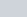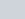There are two temples, one on each bank of a river, just opposite to each other. One temple is 50 m high.
Question:

There are two temples, one on each bank of a river, just opposite to each other. One temple is 50 m high. From the top of this temple, the angles of depression of the top and the foot of the other temple are 30° and 60° respectively. Find the width of the river and the height of the other temple.

Solution:

Letandare two temples each at the bank of river. The top of the temple CE makes angle of depressions at the top and bottom of tower AB are 30° and 60°

Let $C E=50 \mathrm{~m}$ and $A B=H \mathrm{~m}$ and $\angle C B E=60^{\circ}, \angle D A E=30^{\circ}$

The corresponding figure is as follows$\ln \triangle A D E$

$\Rightarrow \quad \tan 30^{\circ}=\frac{h}{x}$

$\Rightarrow \quad \frac{1}{\sqrt{3}}=\frac{h}{x}$

$\Rightarrow \quad x=h \sqrt{3}$

Again in $\triangle B C E$,

$\Rightarrow \quad \tan 60^{\circ}=\frac{50}{x}$

$\Rightarrow \quad \sqrt{3}=\frac{50}{x}$

$\Rightarrow \quad 50=\sqrt{3} \times h \sqrt{3}$

$\Rightarrow \quad h=\frac{50}{3}$

Now the distance between the temples

$x=h \sqrt{3}$

$=\frac{50}{3} \times \sqrt{3}$

$=\frac{50}{\sqrt{3}}$

Therefore $H=50-\frac{50}{3}$

$\Rightarrow \quad H=33.33$

Hence distance between the temples is $\frac{50}{\sqrt{3}} \mathrm{~m}=28.83 \mathrm{~m} \mathrm{~m}$ and height of temple is $33.33 \mathrm{~m}$.